# The displacement (in centimeters) of a particle moving back and forth along a straight line is...

The displacement (in centimeters) of a particle moving back and forth along a straight line is given by the equation of motion s = 5 sin πt + 2 cos πt, where t is measured in seconds. (Round your answers to two decimal places.)

(a) Find the average velocity during each time period.

(i) [1, 2]

? cm/s

(ii) [1, 1.1]

? cm/s

(iii) [1, 1.01]

?cm/s

(iv) [1, 1.001]

?cm/s

(b) Estimate the instantaneous velocity of the particle when t = 1

? cm/s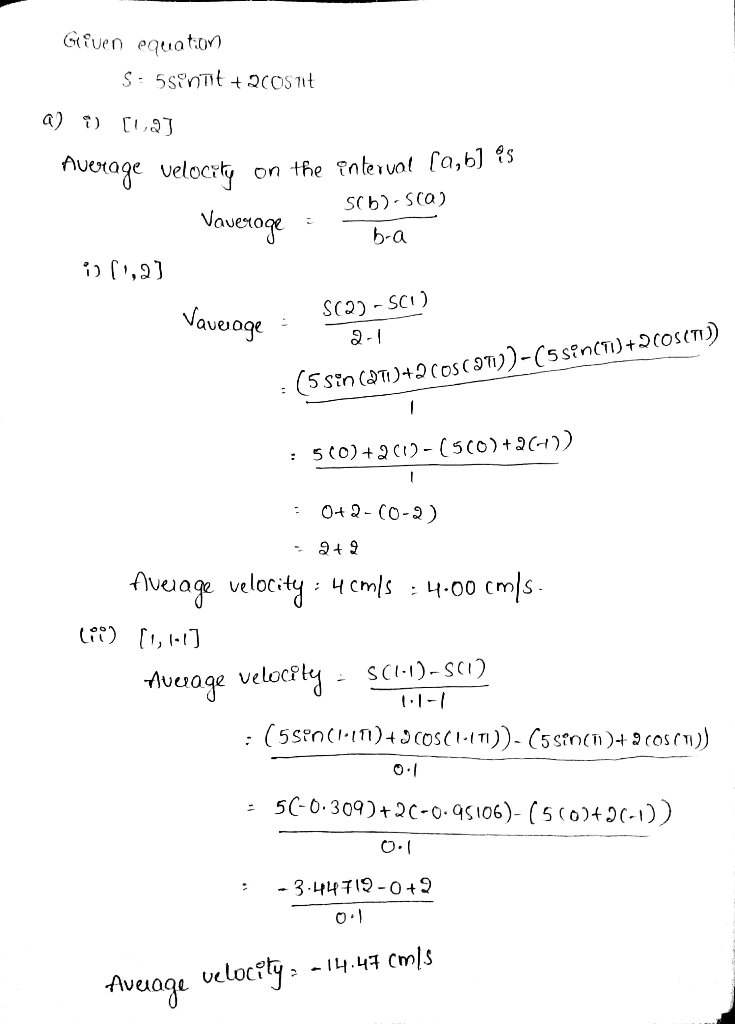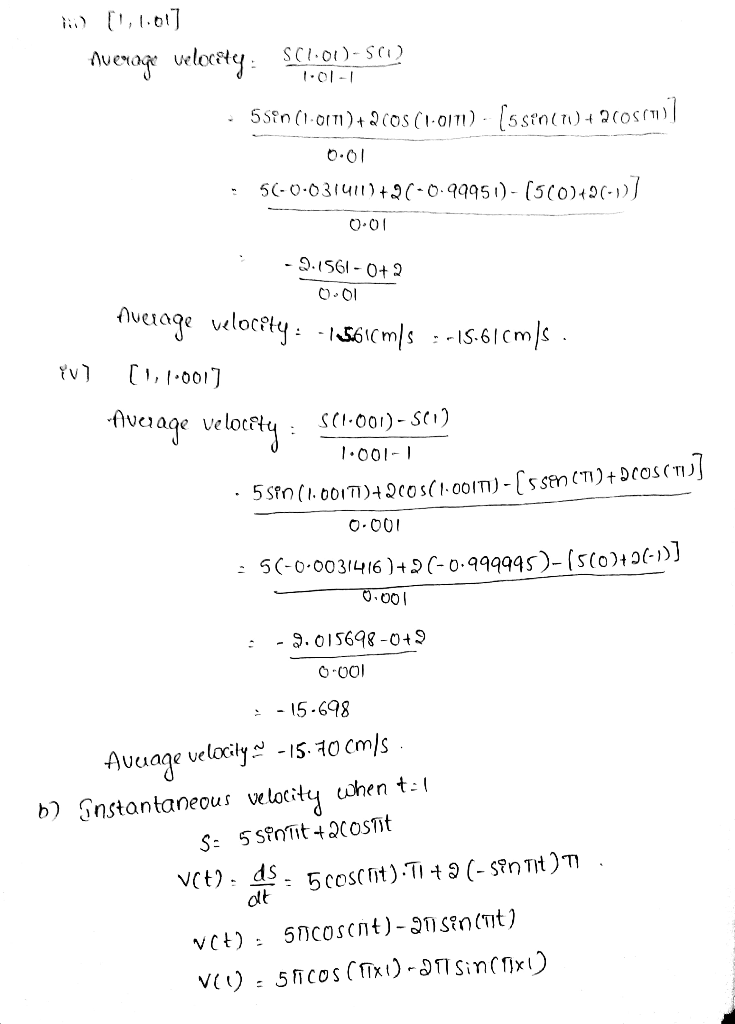#### Earn Coin

Coins can be redeemed for fabulous gifts.

Similar Homework Help Questions
• ### The displacement (in centimeters) of a particle moving back and forth along a straight line is given by the equation of motion s = 4 sin длt + 3 cos длt, where t is measured in seconds

The displacement (in centimeters) of a particle moving back and forth along a straight line is given by the equation of motion s = 4 sin длt + 3 cos длt, where t is measured in seconds. (a)Find the average velocity during each time period. (i) [1, 2](ii) [1, 1.1](iii) [1, 1.01](iv) [1, 1.001](b) Estimate the instantaneous velocity of the particle when t = 1.

• ### The position of a particle moving along the x axis is given in centimeters by x...

The position of a particle moving along the x axis is given in centimeters by x = 9.55 + 1.01 t3, where t is in seconds. Calculate (a) the average velocity during the time interval t = 2.00 s to t = 3.00 s; (b) the instantaneous velocity at t = 2.00 s; (c) the instantaneous velocity at t = 3.00 s; (d) the instantaneous velocity at t = 2.50 s; and (e) the instantaneous velocity when the particle is...

• ### The position of a particle moving along the x axis is given in centimeters by x...

The position of a particle moving along the x axis is given in centimeters by x = 9.79 + 1.97 t3, where t is in seconds. Calculate (a) the average velocity during the time interval t = 2.00 s to t = 3.00 s; (b) the instantaneous velocity at t = 2.00 s; (c) the instantaneous velocity at t = 3.00 s; (d) the instantaneous velocity at t = 2.50 s; and (e) the instantaneous velocity when the particle is...

• ### The position of a particle moving along the x axis is given in centimeters by x...

The position of a particle moving along the x axis is given in centimeters by x = 9.42 + 1.25 t3, where t is in seconds. Calculate (a) the average velocity during the time interval t = 2.00 s to t = 3.00 s; (b) the instantaneous velocity at t = 2.00 s; (c) the instantaneous velocity at t = 3.00 s; (d) the instantaneous velocity at t = 2.50 s; and (e) the instantaneous velocity when the particle is...

• ### A particle moves along a straight a) The average velocity on the line with equation of...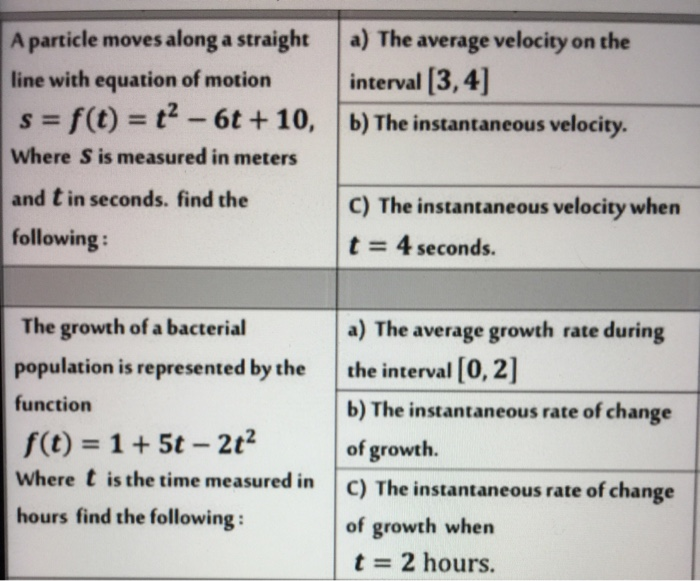A particle moves along a straight a) The average velocity on the line with equation of motion interval [3,4] s= f(t) = t? - 60 + 10, b) The instantaneous velocity. Where S is measured in meters and t in seconds. find the C) The instantaneous velocity when following: t = 4 seconds. The growth of a bacterial population is represented by the function f(t) = 1 + 5t - 2t2 Where t is the time measured in hours find...

• ### Moving along a straight line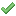The position of a particle moving along the x axis isgiven in centimeters by x = 9.75 + 2.50t3, where t is in seconds.Consider the timeinterval t = 2.00 s to t = 3.00 s.(a) Calculate the average velocity. cm/s(b) Calculate the instantaneous velocity at t = 2.00s. cm/s(c) Calculate the instantaneous velocity at t = 3.00s. cm/s(d) Calculate the instantaneous velocity at t = 2.50s. cm/s(e) Calculate the instantaneous velocity when the particle ismidway between its positions at t...

• ### The position of a particle moving along the x axis is given in centimeters by x...

The position of a particle moving along the x axis is given in centimeters by x = 7.00 + 2.00t3, where t is in seconds. Consider the time interval t = 2.00 s to t = 3.00 s. (Indicate the direction with the sign of your answer.) e) Calculate the instantaneous velocity when the particle is midway between its positions at t = 2.00 s and t = 3.00 s.

• ### The displacement of a particle on a vibrating string is given by the equation s(t) =...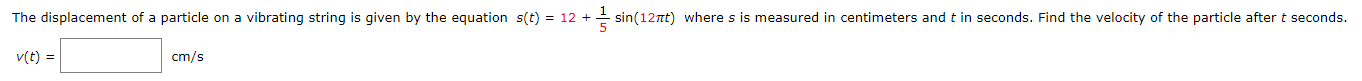The displacement of a particle on a vibrating string is given by the equation s(t) = 12 + * sin(1211) where s is measured in centimeters and t in seconds. Find the velocity of the particle after t seconds. (t) = cm/s

• ### 5.89 kg block oscillates back and forth along a straight line on a frictionless horizontal surface....

5.89 kg block oscillates back and forth along a straight line on a frictionless horizontal surface. Its displacement from the origin is given by x = (20.01 cm) cos((12.74 rad/s)t + 5.89 rad). What is the maximum speed acquired by the block? (Note: Calculate your answer in SI units and do not write the units or unit vectors in the answer box.)

• ### average velocity and instantaneous velocity

The displacement (in centimeters) of a particle moving back andforth along a straight line is given by the equation of motions = 2sin(pt) + 2cos(pt), where tismeasured in seconds. (Round all answers to the nearesthundredth.)(a) Find the average velocity during the time period [1, 2].(b) Find the average velocity during the time period [1, 1.1].(c) Find the average velocity during the time period [1, 1.01].(d) Find the average velocity during the time period [1,1.001].(e) Estimate the instantaneous velocity of the...

Free Homework App